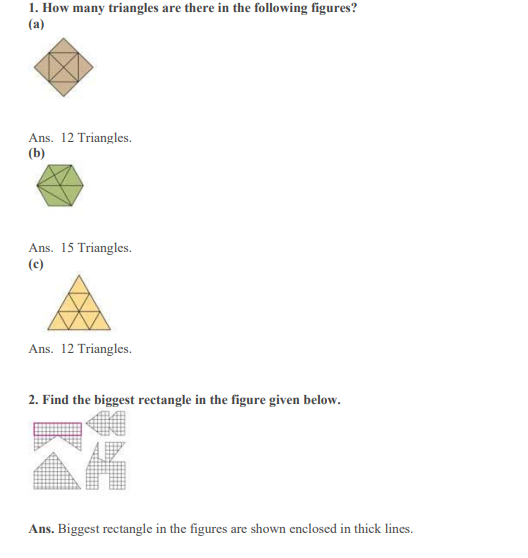# NCERT Solutions Class 3 Mathematics Chapter 5 Shapes and Designs

## Chapter 5 Shapes and Designs Class 3 NCERT Solutions

Class 3 Mathematics students should refer to the following NCERT questions with answers for Chapter 5 Shapes and Designs in standard 3. These NCERT Solutions with answers for Grade 3 Mathematics will come in exams and help you to score good marks

### Chapter 5 Shapes and Designs NCERT Solutions Class 3

NCERT Solutions Class 3 Mathematics Chapter 5 Shapes and Designs. The NCERT solutions for class 3 maths have been made by maths teacher of one of the best CBSE school in India. These NCERT solutions have been made to give detailed answers and expanations of the concepts which can be easily understood by the students. Refer to other links also to download mathematics NCERT solutions, worksheets, sample papers and test papers.## Books recommended by teachers

 NCERT Solutions Class 3 Mathematics Chapter 1 Where to Look from
 NCERT Solutions Class 3 Mathematics Chapter 2 Fun with numbers
 NCERT Solutions Class 3 Mathematics Chapter 3 Give and Take
 NCERT Solutions Class 3 Mathematics Chapter 4 Long and Short
 NCERT Solutions Class 3 Mathematics Chapter 5 Shapes and Designs
 NCERT Solutions Class 3 Mathematics Chapter 6 Fun with Give and Take
 NCERT Solutions Class 3 Mathematics Chapter 7 Times go On
 NCERT Solutions Class 3 Mathematics Chapter 8 Who is heavier
 NCERT Solutions Class 3 Mathematics Chapter 9 How many times
 NCERT Solutions Class 3 Mathematics Chapter 10 Play with Patterns
 NCERT Solutions Class 3 Mathematics Chapter 11 Jugs and Mugs
 NCERT Solutions Class 3 Mathematics Chapter 12 Can we share
 NCERT Solutions Class 3 Mathematics Chapter 13 Smart Charts
 NCERT Solutions Class 3 Mathematics Chapter 14 Rupees and Paise